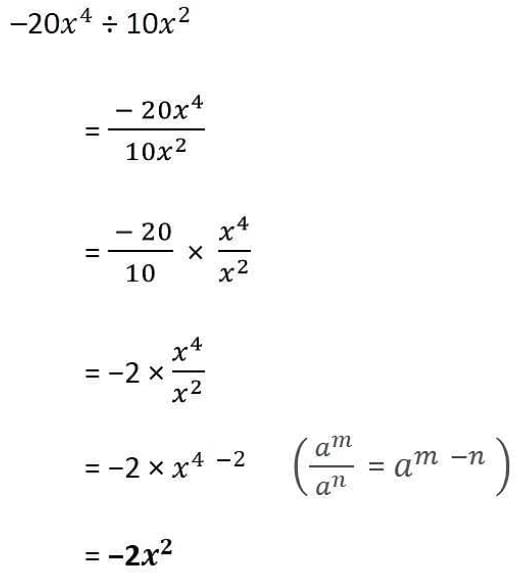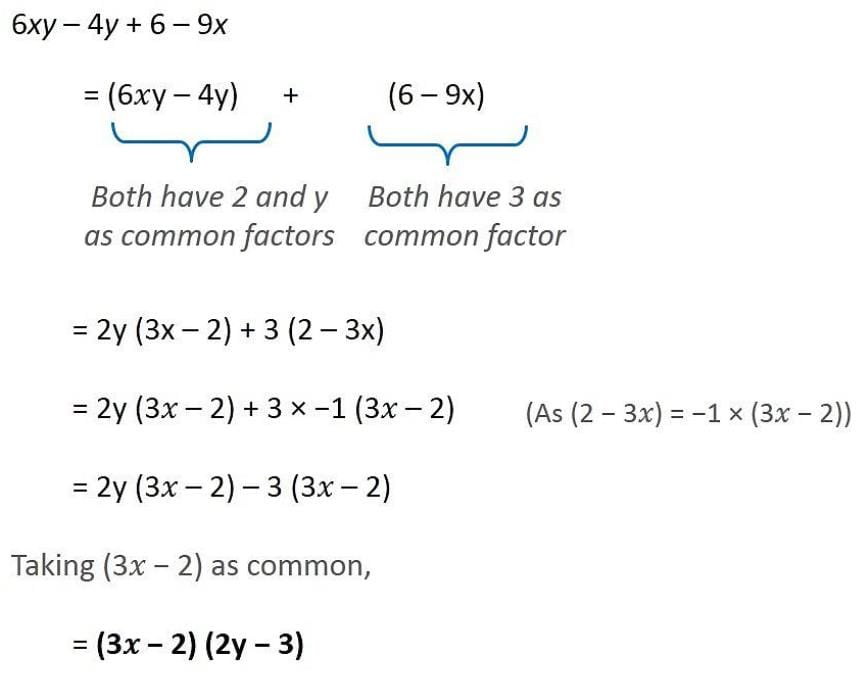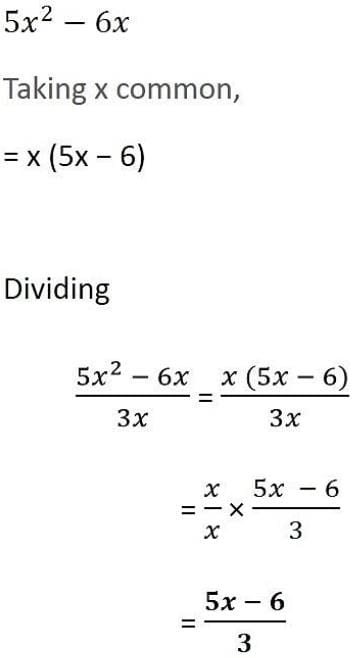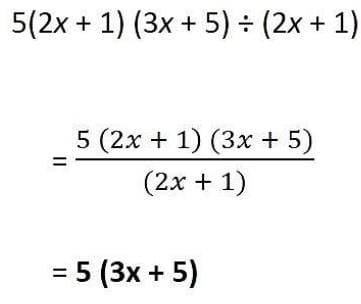# Test: Factorisation- 2

## 10 Questions MCQ Test Mathematics (Maths) Class 8 | Test: Factorisation- 2

Description
Attempt Test: Factorisation- 2 | 10 questions in 10 minutes | Mock test for Class 8 preparation | Free important questions MCQ to study Mathematics (Maths) Class 8 for Class 8 Exam | Download free PDF with solutions
QUESTION: 1

### Factorise 12a2b+ 15ab2

Solution:

Now, 12a2 b + 15ab2

= (3 * 2 * 2 * a * a * b) + (3 * 5 * a * b * b)

= (3 * a * b) {(2 *2 * a) + (5 * b)}

= 3ab (4a + 5b)

QUESTION: 2

### Factorise: x2+ 8x + 16

Solution:

C ) (x^2 +8x +16) = (x^2 + 4x ×4x + 16) = ( x^2 + 4x )(4x + 16 ) = x(x + 4 ) 4(x + 4 ) =(x + 4)(x + 4) =(x + 4)^2 OR(x^2 +8x + 16)(x^2 +2 × x × 4x + 4^2)(x + 4)^2

QUESTION: 3

### Solve: –20(x)4 ÷ 10(x)2

Solution:QUESTION: 4

When we factorise an expression, we write it as a ________ of factors.

Solution:

When we factorise an algebraic expression, we write it as a product of factors. These factors may be numbers, algebraic variables or algebraic expressions.

QUESTION: 5

Factorise 6xy – 4y + 6 – 9x.

Solution:QUESTION: 6

Factorize x² + 8x + 12

Solution:

Factors of 12x are 2*2*3*x and factors of 36 are 2*2*3*3. Hence the common factors are2*2*3 =12.

QUESTION: 7

Which of the following is quotient obtained on dividing –18 xyz2 by –3 xz?

Solution:

x and z gets cancelled ,-3 gets cancelled . So the quotient obtained is 6yz

QUESTION: 8

Divide the given polynomial by the given monomial: (5x2− 6x) ÷ 3x

Solution:QUESTION: 9

Divide as directed: 5 (2x + 1) (3x + 5) ÷ (2x + 1)

Solution:QUESTION: 10

Factorise: p4– 81

Solution:
We have  p4 - 81 = (p^2)^2 - (9^2)^2

Now using a^2 - b^2 = (a + b)(a - b), we have

(p^2)^2 - (9)^2 = (p^2 + 9)(p^2 -9)

We can factorise p^2 - 9  further as
p^2 - 9 = (p)^2 - (3)^2

= (p + 3)(p - 3)

∴           p^4 - 81 = (p + 3)(p - 3)(p^2 + 9)Use Code STAYHOME200 and get INR 200 additional OFF Use Coupon Code